{{announcement.body}}
{{announcement.title}}

# Simple Linear Regression Model: How to Use R Deducer Package

DZone 's Guide to

# Simple Linear Regression Model: How to Use R Deducer Package

### A Java-based GUI, Deducer competes with its rivals like SAS and SPSS without compromising on the quality of output. Learn how it aids linear models.

· Big Data Zone ·
Free Resource

Comment (5)

Save
{{ articles.views | formatCount}} Views

Linear models — better known as linear regression — are one of the most common and flexible analysis frameworks to identify relationships between two or more variables. The widely used linear model is represented by drawing the best-fit line through a series of data points represented on a scatter plot.

For any budding business analyst, this is a good starting point to understand how the model works at the very core of its design.

Selecting the variables in the Deducer GUI:

• Outcome variable: Y, or the dependent variable, should be put on this list
• As numeric: Independent variables that should be treated as covariates should be put in this section. Deducer automatically converts a factor into a numeric variable, so make sure that the order of the factor level is correct
• As factor: Categorically independent variables (language, ethnicity, etc.).
• Weights: This option allows the users to apply sampling weights to the regression model.
• Subset: Helps to define if the analysis needs to be done within a subset of the whole dataset.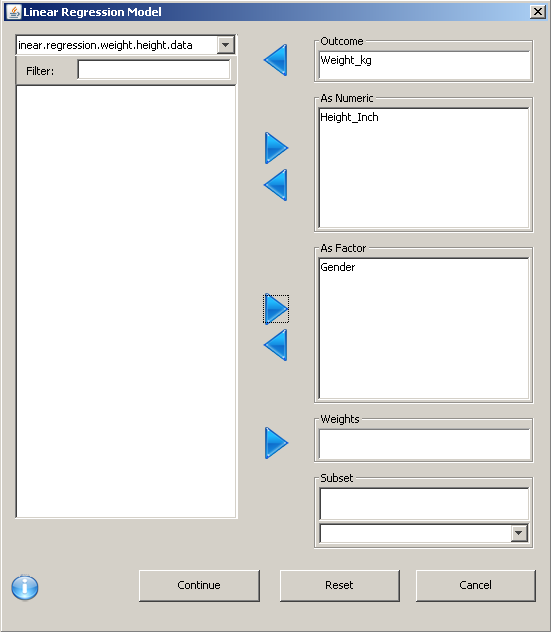Note: Only one outcome is allowed. It can also be transformed by double-clicking on it. For example, the log transform weight for the analysis can be changed to log(weight).

## Model Tab

Users can add terms to the model by selecting one or more variables from the variable list.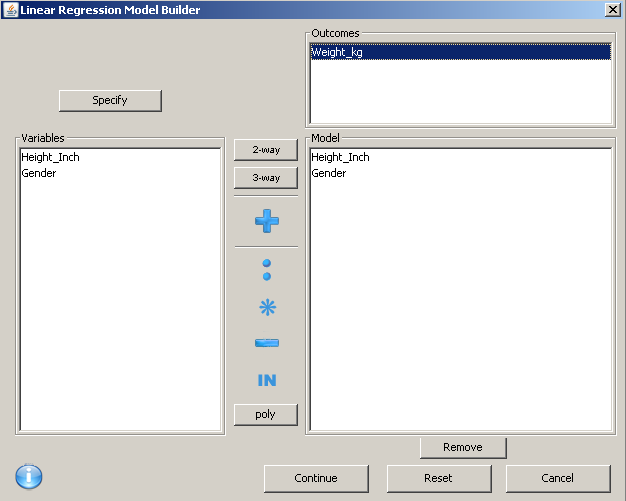• 2-way: Add all two-way and lower interactions between the selected variables.

• 3-way: Add all three-way and lower interactions between the selected variables.

• + — Add main effects for all the selected variables

• : — Add interaction between selected models

• *  Add interaction in between the selected terms, as well as any lower order interactions with them

• -  Remove term

• Poly: Add orthogonal polynomial terms to the model

## Exploring the Model

After model creation, using this tab, the features of the model can be explored. The Preview panel displays a preview of what will be displayed in the console when the model is run. In the upper left-hand portion of the dialog, there are icons representing the assumptions that are being made by the model.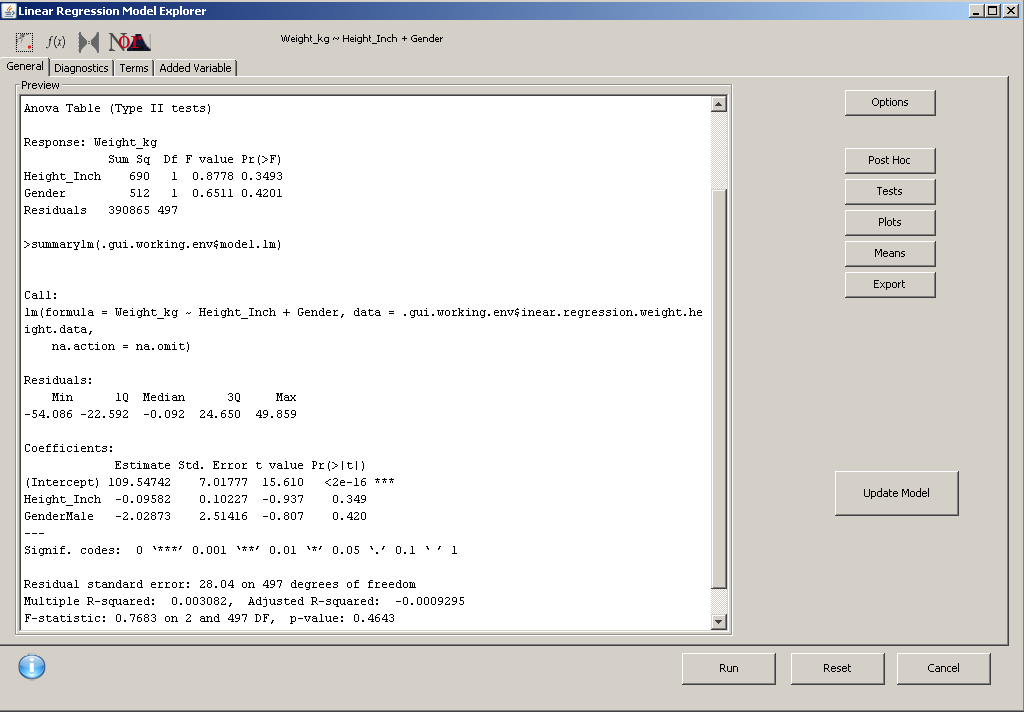The above interactive console provides the following options to perform some detailed analysis:

• Option: This controls the main tests and diagnostic summaries of the model.
• ANOVA table
• Summary table
• Unequal variance
• Diagnostics, i.e. VIF (variance inflation factors), influence summary
• Post hoc: Helps to compare the levels of factors.
• Post hoc: The factors for which it should be calculated
• Type: Comparison type, i.e. Tukey does all the pairwise comparisons
• Estimate CI: Should confidence intervals be calculated?
• Corrections: Correct the p-values and CI, if the factor has >2 levels
• Tests: Customer hypothesis test based on the model parameters.
• Plots: Visualize the marginal effects of the model.
• Pointwise intervals: Plot pointwise CI
• Y-axis labels: Labels for the y-axis plots
• Multiple lines per panel: If the effect is an interaction effect, this option decides whether the interaction should be plotted on multiple lines within the same panel or as separate panels
• Rug: Small lines on the x-axis denoting the data distributions
• # of levels: Number of levels for which the effect should be calculated
• Means (marginal means): Just like the effects plots, the marginal means are the estimated means based on the model’s outcome variable across the levels of termsl given the other terms are static or at the typical level.
• Export: Linear model export allows users to export a number of relevant variables related to the model.

## Diagnostic Tab

This panel contains six plots evaluating the outlier, influence, and equality of variance.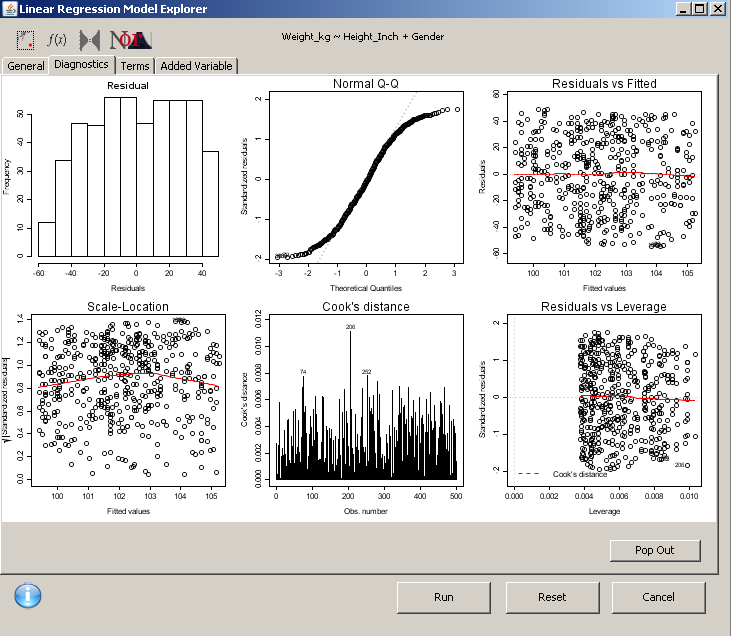The above two plots show the distribution of the residuals and ideally, these should be normal.

Residual vs. fitted: Shows the residuals of the model plotted against the predicted values. If the red line is not flat, then the model may have significant non-linearity.

Scale location: Plots the predicted values vs. the square root of the standardized residuals; also known as spread vs. level.

Cooks distance: Linear model is sensitive to outliers that can unduly influence the results of the model. Therefore, Cooks distance helps the analysts to identify observations with Cooks values that are greater than 1.

Residuals vs. leverage: Another plot to examine outliers and influence.

Term plots: Also known as component or partial residual plots.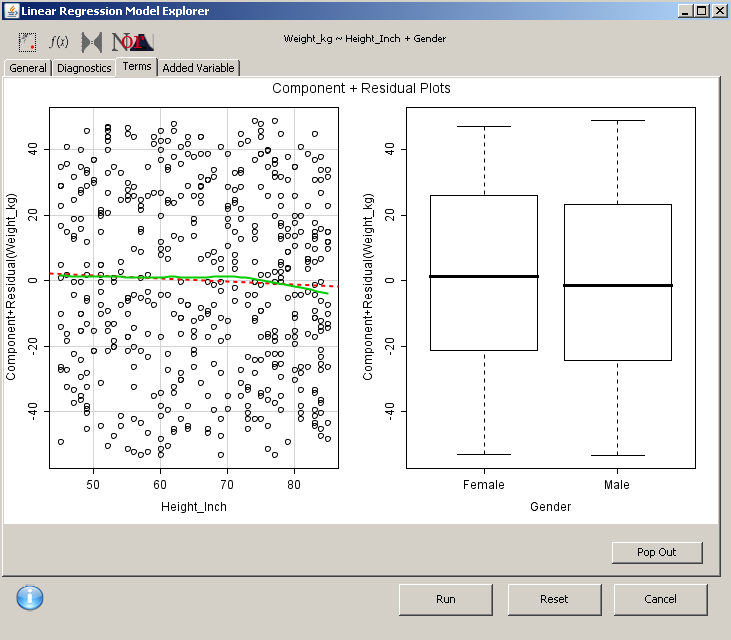For models without interactions, component residual plots are given. These can be used to examine the linearity of the relationship between the predictor and outcome variables.

• For numeric variables, a scatter plot is produced.
• For factors, a box plot is generated.

Just like plots, added variable plots are used to examine the linearity of covariates. They are highly recommended when there are no term plots available.

In a nutshell, Deducer is one of the most functional GUIs with mass appeal. The ease of use that Deducer offers to its users is second to none. Deducer continues to amaze everyone by accepting file formats for leading statistical software, like:

• Minitab
• SPSS
• SAS
• Dbase
• Excel

Being a Java-based GUI, it competes with its rivals like SAS and SPSS without compromising on the quality of output. Especially for businesses and individuals with tight budgets, Deducer can be deployed without spending hundreds and thousands of dollars.

Topics:
big data, data analytics, data visualization, deducer gui, linear regression, r, tutorial

Comment (5)

Save
{{ articles.views | formatCount}} Views

Published at DZone with permission of Sunil Kappal , DZone MVB. See the original article here.

Opinions expressed by DZone contributors are their own.